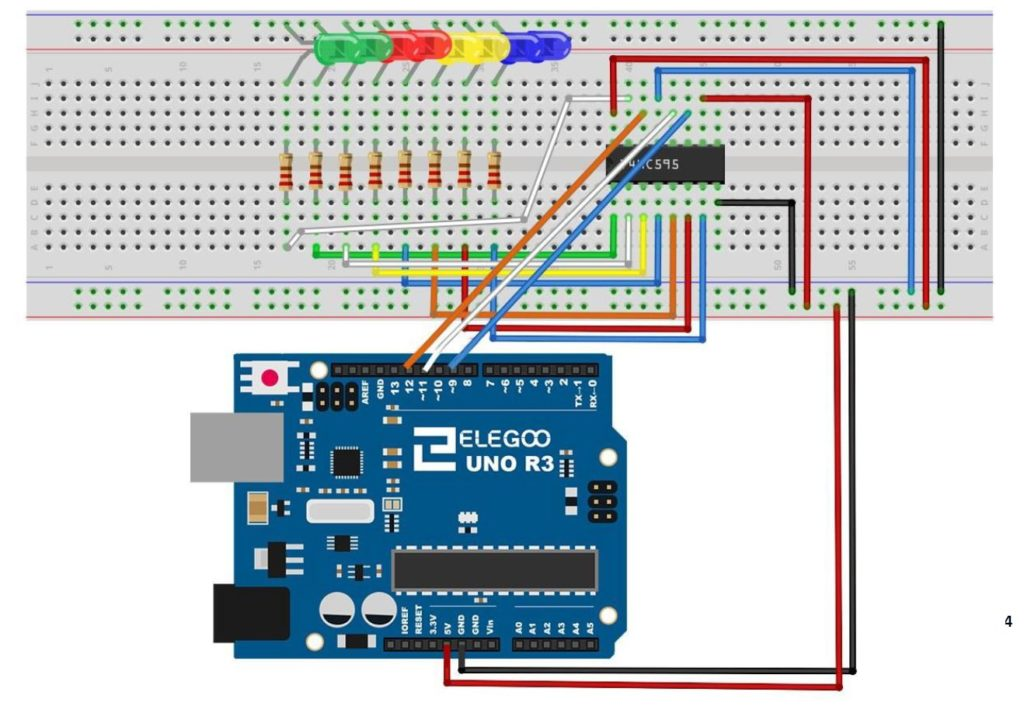# Arduino Tutorial 46: HOMEWORK- Create a Binary Bit Flipper with the 74HC595

The purpose of this lesson is to assign you homework. Your homework is to create a Bit Flipper. That is, for an 8 bit Binary or Hex number, invert the bits . . . “1” bits should become “0” and “0” bits should become “1”. For example,

if myByte=00001111

the flipped version of this would be

myByteFlipped=11110000

Similarly if myByte=00000001

myByteFlipped=11111110

You could do this with 255 if statements, but see if you can figure out a better way of doing it, and then demonstrate your results using the circuit we have been using in the last few lessons.This is the schematic we use in this example to control 8 LEDs from the 74HC595 chip.